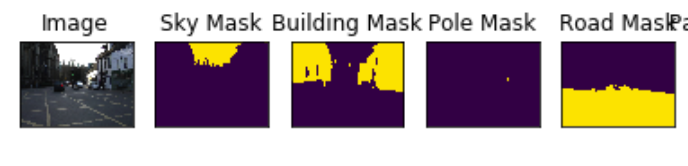# How to define the order of mask class names in PyTorch data loader?

The following data loader script reads 11 different class names from ‘mask’ images. It seems that the index of the classes is used to define the order. But, when you have an image that has 11 different mask-classes with no names assigned, how could the first index be a ‘sky’ and second could be ‘building’ and so on? I am having a hard time understanding this logic.

``````class Dataset(BaseDataset):
"""CamVid Dataset. Read images, apply augmentation and preprocessing transformations.

Args:
images_dir (str): path to images folder
class_values (list): values of classes to extract from segmentation mask
augmentation (albumentations.Compose): data transfromation pipeline
(e.g. flip, scale, etc.)
preprocessing (albumentations.Compose): data preprocessing
(e.g. normalization, shape manipulation, etc.)

"""

CLASSES = ['sky', 'building', 'pole', 'road', 'pavement',
'tree', 'signsymbol', 'fence', 'car',
'pedestrian', 'bicyclist', 'unlabelled']

def __init__(
self,
images_dir,
classes=None,
augmentation=None,
preprocessing=None,
):
self.ids = os.listdir(images_dir)
self.images_fps = [os.path.join(images_dir, image_id) for image_id in self.ids]

# convert str names to class values on masks
self.class_values = [self.CLASSES.index(cls.lower()) for cls in classes]

self.augmentation = augmentation
self.preprocessing = preprocessing
``````

Example image:It seems the order of the classes is defined in the script using `CLASSES`?
I don’t know what exactly is passed to the `Dataset`, but based on how `self.class_values` is created, it seems to be the case.

The index are in the mask images itself. So for example if the label for “sky” is 1, in the mask images with sky should be full of 1 in the correspondents pixels.

1 Like

You are right. Camvid masks have labels from 1 to 11. In my case, I do not have classes in order and they range from 0 to 23 and these are greyscale images. How to deal with this?

``````Array Dimensions (360, 480)
array([ 0,  1,  2,  3,  4,  5,  6,  7,  8,  9, 10, 11], dtype=uint8)
``````

``````Array Dimensions (512, 512, 3)
array([ 0,  2,  5,  9, 21, 22, 23], dtype=uint8)
``````

You could adapt your data and labels to CamVid (for example using a dictionary to map your labels to CamVid labels) or either create your own Dataset

I am trying to create my own dataset like this but not sure how to assign the class order to the class names.
Is it something like this

``````    dict = {
0: "right_ra",
2: "left_ra",
5: "right_p",
9: "left_p",
21: "right_es",
22: "left_es",
23: "background"
}

CLASSES = pd.DataFrame([dict])
``````

``````from torch.utils.data import DataLoader
from torch.utils.data import Dataset as BaseDataset

class Dataset(BaseDataset):

CLASSES = ['right_ra', 'left_ra',
'right_p', 'left_p', 'right_es',
'left_es', 'background']

def __init__(
self,
images_dir,
classes=None,
augmentation=None,
preprocessing=None,
):
self.ids = os.listdir(images_dir)
self.images_fps = [os.path.join(images_dir, image_id) for image_id in self.ids]

# convert str names to class values on masks
self.class_values = [self.CLASSES.index(cls.lower()) for cls in classes]

self.augmentation = augmentation
self.preprocessing = preprocessing

def __getitem__(self, i):

#image = cv2.cvtColor(image, cv2.COLOR_BGR2RGB)

# extract certain classes from mask

# apply augmentations
if self.augmentation: